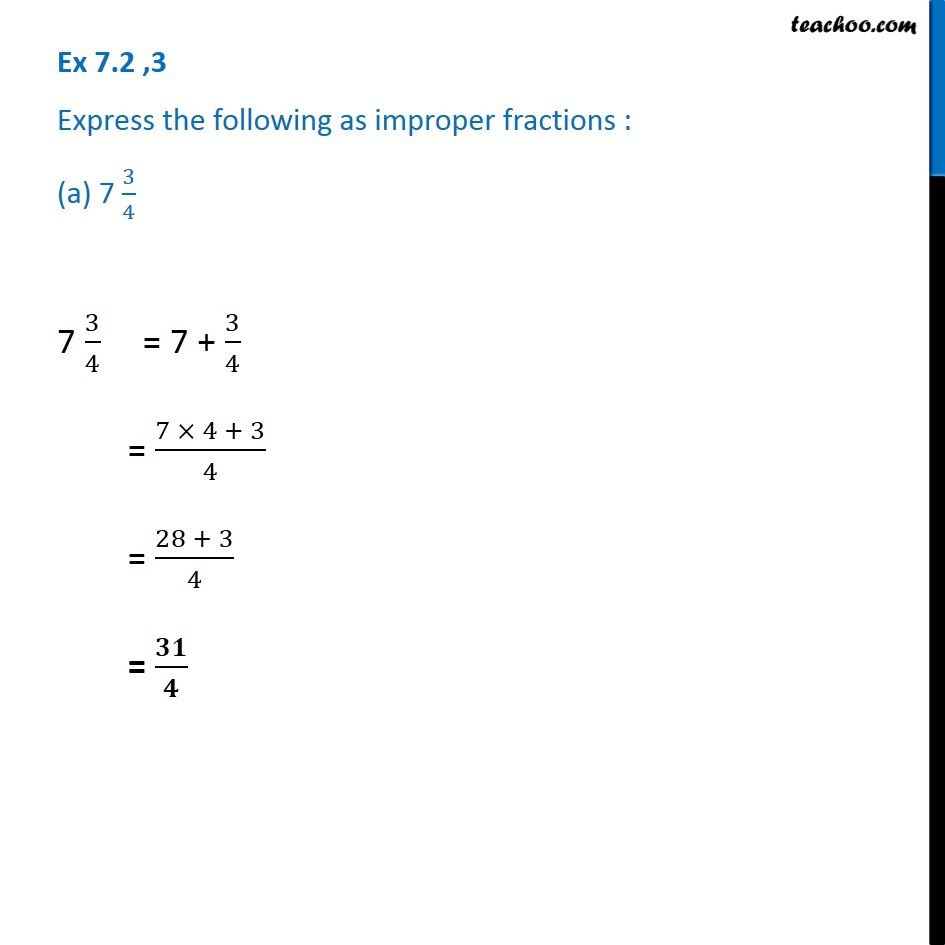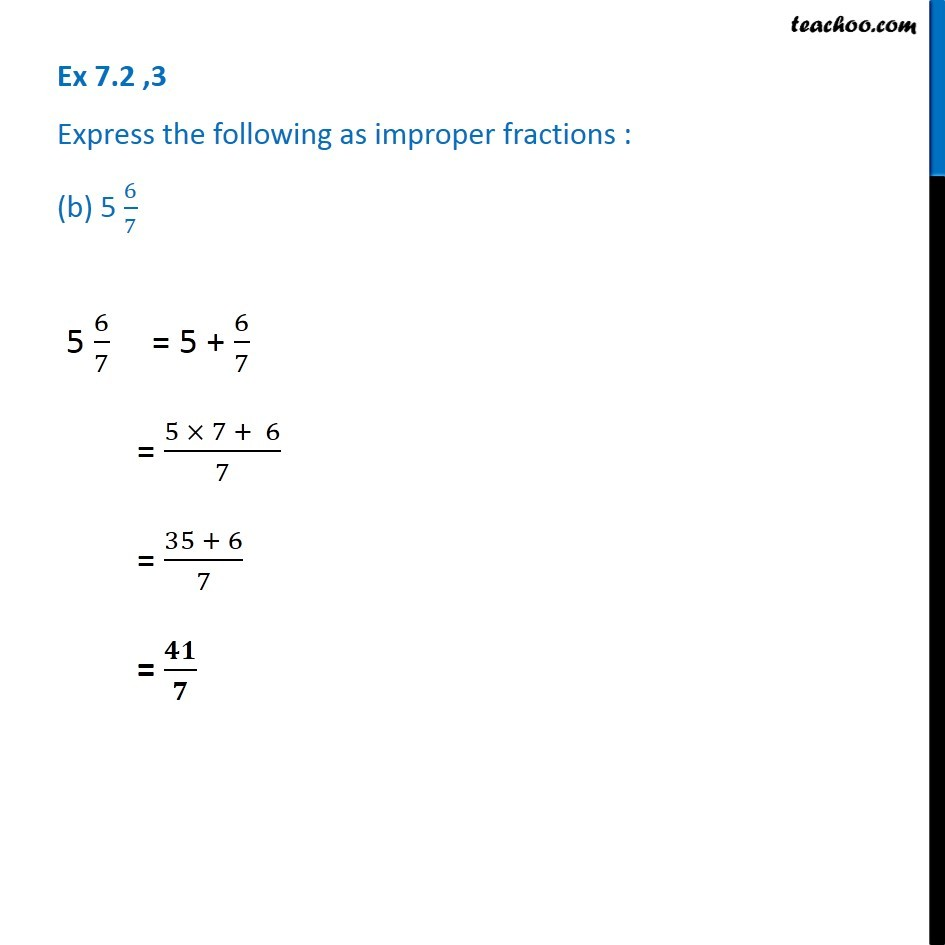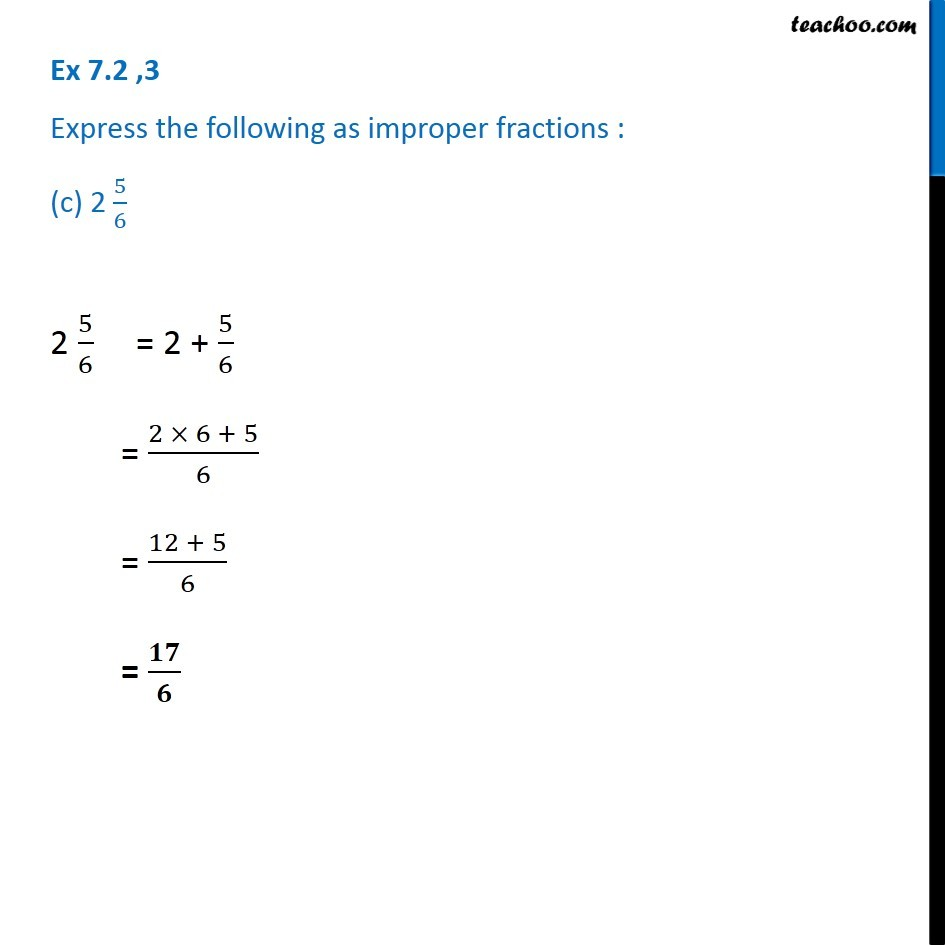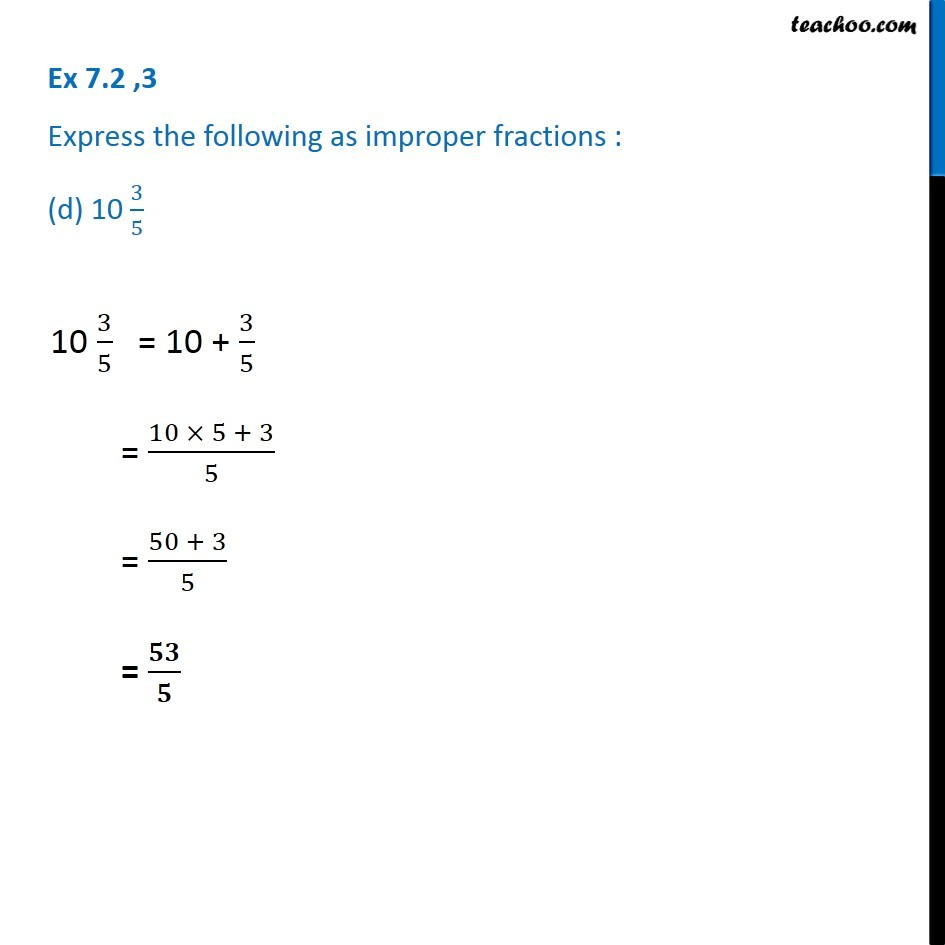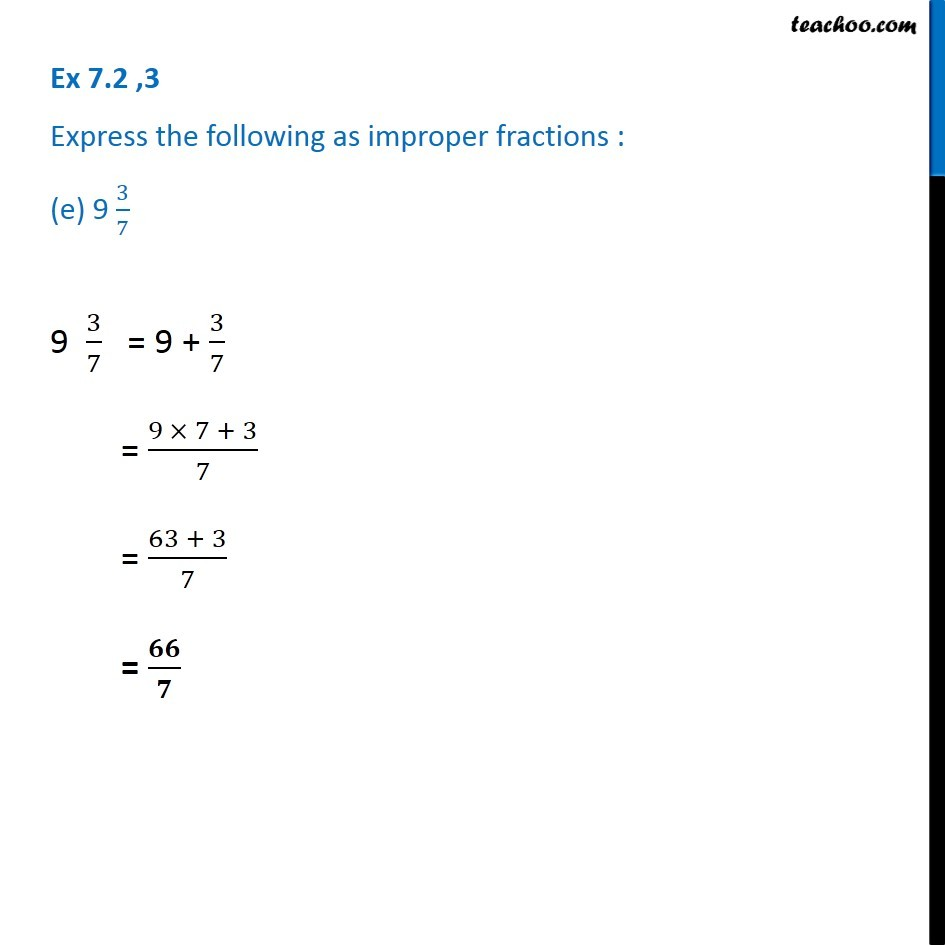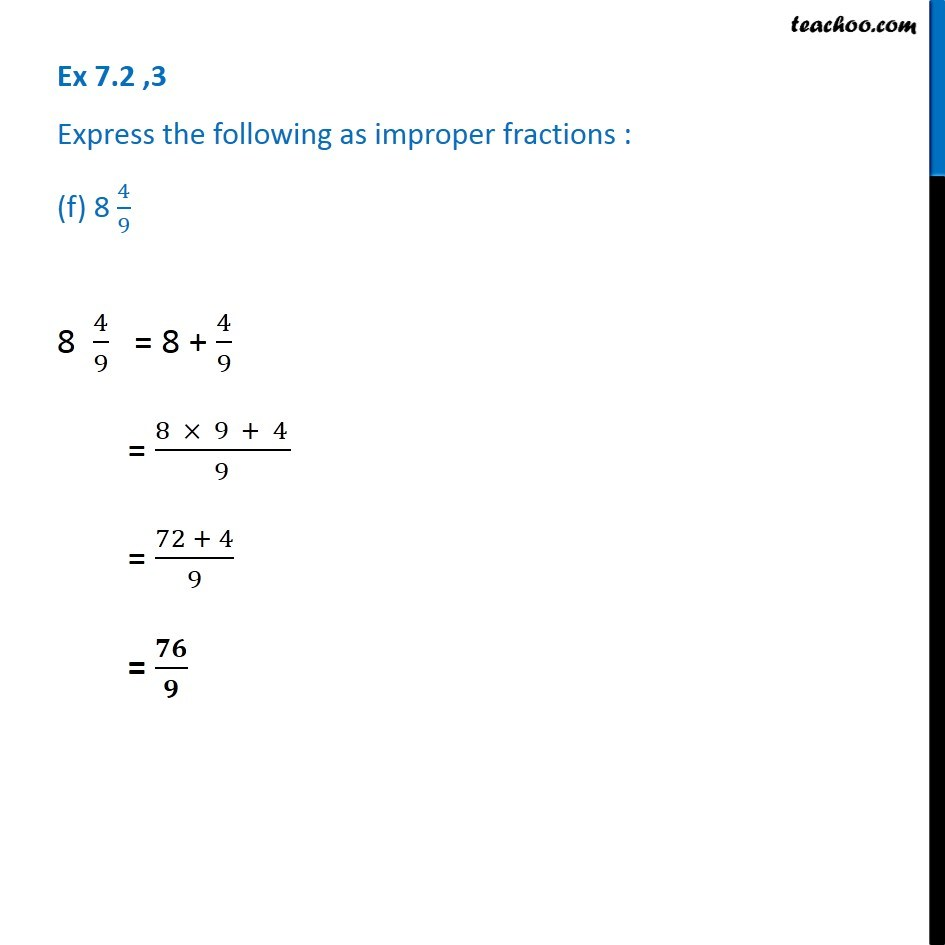Subscribe to our Youtube Channel - https://you.tube/teachoo

1. Chapter 7 Class 6 Fractions
2. Serial order wise
3. Ex 7.2

Transcript

Ex 7.2 ,3 Express the following as improper fractions : (a) 7 3/4 7 3/4 = 7 + 3/4 = (7 × 4 + 3)/4 = (28 + 3)/4 = 𝟑𝟏/𝟒 Ex 7.2 ,3 Express the following as improper fractions : (b) 5 6/7 5 6/7 = 5 + 6/7 = (5 × 7 + 6)/7 = (35 + 6)/7 = 𝟒𝟏/𝟕 Ex 7.2 ,3 Express the following as improper fractions : (c) 2 5/6 2 5/6 = 2 + 5/6 = (2 × 6 + 5)/6 = (12 + 5)/6 = 𝟏𝟕/𝟔 Ex 7.2 ,3 Express the following as improper fractions : (d) 10 3/5 10 3/5 = 10 + 3/5 = (10 × 5 + 3)/5 = (50 + 3)/5 = 𝟓𝟑/𝟓 Ex 7.2 ,3 Express the following as improper fractions : (e) 9 3/7 9 3/7 = 9 + 3/7 = (9 × 7 + 3)/7 = (63 + 3)/7 = 𝟔𝟔/𝟕 Ex 7.2 ,3 Express the following as improper fractions : (f) 8 4/9 8 4/9 = 8 + 4/9 = (8 × 9 + 4)/9 = (72 + 4)/9 = 𝟕𝟔/𝟗

Ex 7.2

Chapter 7 Class 6 Fractions
Serial order wise